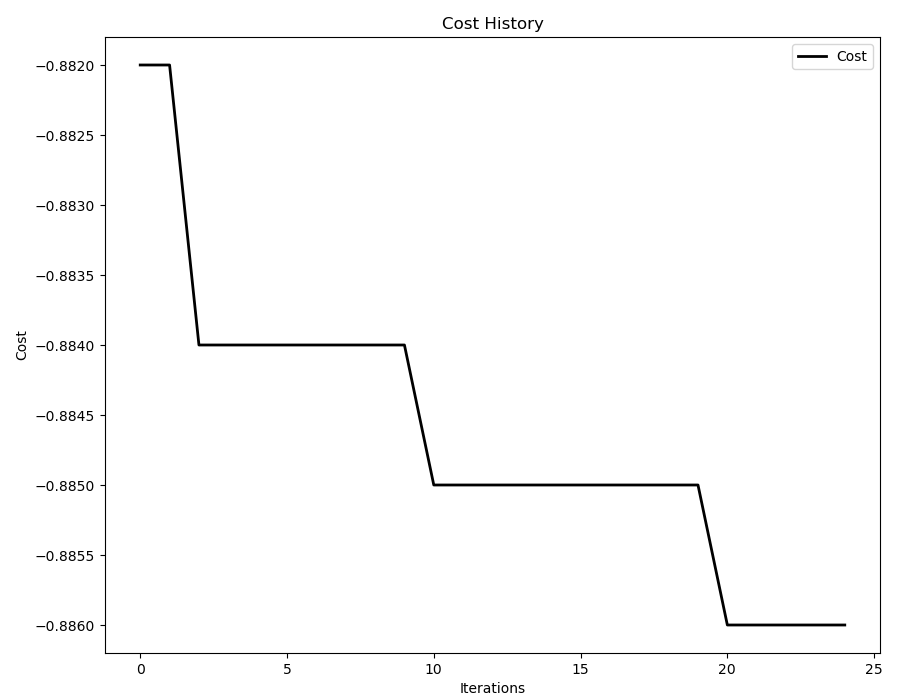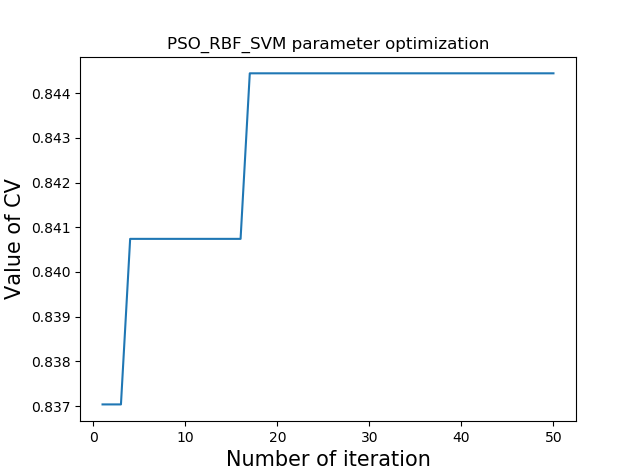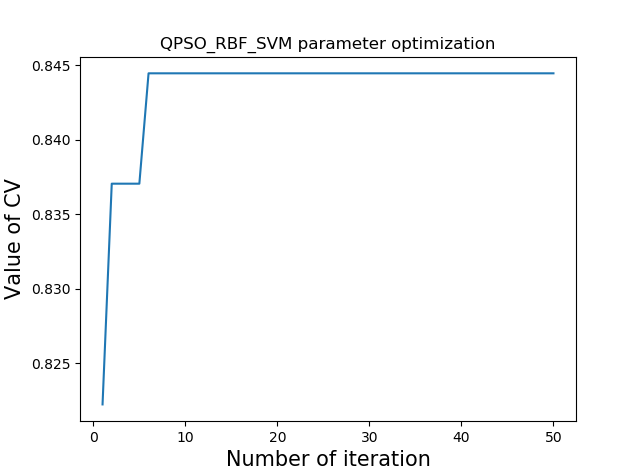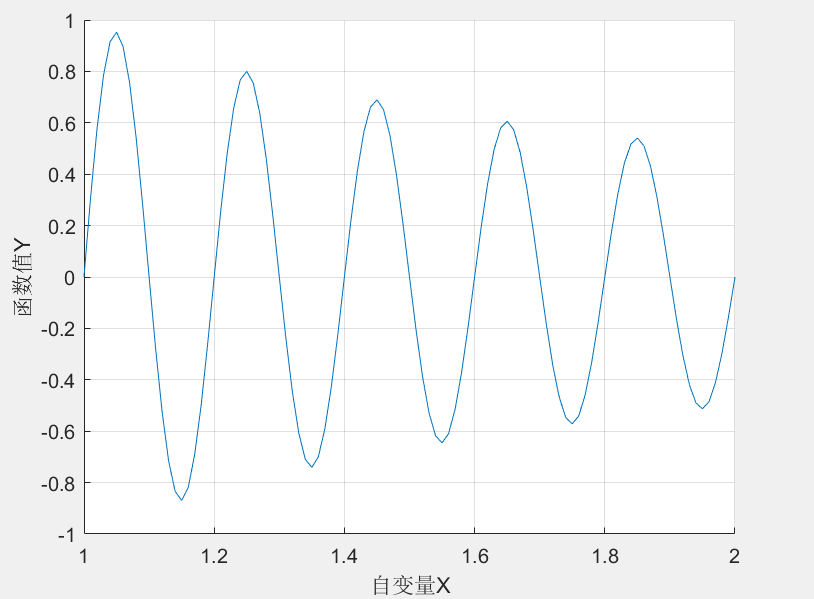• 2022-01-03 15:36:10

相关文章：

## 1 GlobalBestPSO() 函数简介

PSO 超参数调优采用的是 pyswarm 包中的 GlobalBestPSO()

class pyswarms.single.global_best.GlobalBestPSO(n_particles, dimensions, options, bounds=None, oh_strategy=None, bh_strategy=‘periodic’, velocity_clamp=None, vh_strategy=‘unmodified’, center=1.0, ftol=-inf, ftol_iter=1, init_pos=None)

输入参数：

• n_particles：整数，粒子群中的粒子数量
• dimension：整数，空间维度，或特征维度
• options：系数，字典形式 {‘c1’=2, ‘c2’=2, ‘w’=0.3}
• bounds：数组，可选，行数为 2，第一行是边界的最小值，第二行是边界的最大值
• oh_strategy：字典，可选，每个 option 的选择策略
• bh_strategy：字符串，处理超出边界的粒子的策略
• velocity_clamp：元组，可选，一个大小为2的元组，其中第一个条目是最小速度，第二个条目是最大速度。它设定了速度的限制
• vh_strategy：字符串，处理超出边界的粒子速度的策略
• ftol：列表（默认为None），

GlobalBestPSO()的优化方法

optimize(objective_func, iters, n_processes=None, verbose=True, **kwargs)

输入参数：

• objective_func (function)：自适应函数或损失函数
• iters (int)：迭代次数
• kwargs (dict) ：目标函数的参数

返回：

• tuple：目标函数最优值和最优位置

## 2 定义PSO优化器

import numpy as np
import pyswarms as ps
from sklearn.datasets import make_classification
from sklearn.linear_model import LogisticRegression
from sklearn.metrics import confusion_matrix, classification_report
from sklearn.model_selection import cross_val_score

n_particles = 10
cv = 5
options = {'c1': 0.5, 'c2': 0.5, 'w': 0.9}
optimizer = ps.single.GlobalBestPSO(n_particles=n_particles, dimensions=dims,
options=options, bounds=bounds)


## 3 定义参数和边界

这里选择逻辑回归模型的超参数：C 和 penalty。

# 超参数 C 和 penalty
C = [0., 1.]
penalty = ['l1', 'l2', 'elasticnet', 'none']
penalty_bound = [0, len(penalty)]  # 把这个看作索引
dims = 2

# bounds表示所有参数的界限
max_ = np.zeros(dims)
min_ = np.zeros(dims)

for i, param in enumerate([C, penalty_bound]):
min_[i] = param
max_[i] = param

bounds = (min_, max_)
print(bounds)


输出：

(array([0., 0.]), array([1., 4.]))


## 4 得到最优模型

# 这里目标函数的输入为需要优化的参数
best_cost, best_pos = optimizer.optimize(objective, iters=25)
print(best_pos, penalty[int(best_pos)])
best_model = LogisticRegression(C=best_pos, penalty=penalty[int(best_pos)])
best_model = best_model.fit(X_train, y_train)


输出：

[0.01806252 1.430832  ] l2


## 5 定义目标函数

在定义目标函数时，需要将每个粒子的值带入模型中训练，然后返回模型的指标值。这个指标值可以是分类中的准确率。

具体如下：

def objective(param):
"""计算每个粒子的适应度，param表示输入的参数(n_particles, n_dims)"""
scores = np.zeros((n_particles, cv))
clf = LogisticRegression
for i in range(n_particles):
"""计算每个粒子的得分"""
scores[i, :] = cross_val_score(clf(C=param[i], penalty=penalty[int(param[i])]),
X_train, y_train,
scoring='accuracy',
cv=cv)
return -np.mean(scores, axis=1)


## 6 查看损失变化

查看目标函数随迭代增加的变化情况：

from pyswarms.utils.plotters import plot_cost_history
plot_cost_history(cost_history=optimizer.cost_history)## 7 测试模型

X, y = make_classification(n_samples=2000, n_features=5, random_state=42)

X_train, y_train = X[:1000], y[:1000]
X_test, y_test = X[1000:], y[1000:]

y_pred = best_model.predict(X_test)
print(confusion_matrix(y_test, y_pred))
print(classification_report(y_test, y_pred))


输出：

[[440  55]
[ 45 460]]
precision    recall  f1-score   support
0       0.91      0.89      0.90       495
1       0.89      0.91      0.90       505
accuracy                           0.90      1000
macro avg       0.90      0.90      0.90      1000
weighted avg       0.90      0.90      0.90      1000分类 数据挖掘 python
更多相关内容
• 【寻优算法】粒子群算法（PSO） 参数寻优的python实现一、算法原理1、粒子群算法的名词解释2、粒子更新二、PSO算法参数寻优的python实现参考资料 粒子群优化算法（Particle swarm optimization，PSO）是模拟鸟群...

### 【超参数寻优】粒子群算法（PSO） 超参数寻优的python实现

粒子群优化算法（Particle swarm optimization，PSO）是模拟鸟群捕食行为的优化算法。不同于遗传算法（Genetic Alogrithm，GA），粒子群算法是有记忆的，之前迭代过程中的最优位置和最优方向都会保留下来并作用于粒子群的更新【参考资料1】。

# 一、算法原理

## 1、粒子群算法的名词解释

粒子群长度：粒子群长度等于每一个参数取值范围的大小。
粒子群维度：粒子群维度等于待寻优参数的数量。
粒子群位置：粒子群位置包含参数取值的具体数值。
粒子群方向：粒子群方向表示参数取值的变化方向。
适应度函数：表征粒子对应的模型评价指标。
pbest:（局部最优）pbest的长度等于粒子群长度，表示每一个参数取值的变化过程中，到目前为止最优适应度函数值对应的取值。
gbest:（全局最优）gbest的长度为1，表示到目前为止所有适应度函数值中最优的那个对应的参数取值。
惯性因子 w w ：惯性因子表示粒子保持的运动惯性。
局部学习因子 c 1 {c_1} ：表示每个粒子向该粒子目前为止最优位置运动加速项的权重。
全局学习因子 c 2 {c_2} ：表示每个粒子向目前为止全局最优位置运动加速项的权重。

## 2、粒子更新

粒子方向更新方程为：

v i = w × v i + c 1 × r a n d ( ) × ( p b e s t i − x i ) + c 2 × r a n d ( ) × ( g b e s t − x i ) {v_i} = w \times {v_i} + {c_1} \times rand() \times (pbes{t_i} - {x_i}) + {c_2} \times rand() \times (gbest - {x_i})

其中 v i {v_i} 表示第i个粒子的方向， p b e s t i pbes{t_i} 表示第i个粒子当前最优参数， g b e s t gbest 表示当前的全局最优参数， x i x_i 表示第i个粒子的参数值， r a n d ( ) rand() 表示介于 ( 0 , 1 ) (0,1) 之间的随机数。

粒子位置更新方程为：

x i = x i + v i {x_i} = {x_i} + {v_i}

w w 取值越大说明粒子更新更多受到粒子历史方向的影响， w = 0 w=0 表示粒子更新只取决于粒子当前位置，局部最优粒子位置和全局最优粒子位置，粒子方向本身没有记忆性。 c 1 {c_1} 表示粒子更新受到局部最优粒子的影响程度， c 1 = 0 {c_1}=0 表示粒子只有全局搜索能力，但缺少局部搜索能力。 c 2 {c_2} 表示粒子更新受到全局最优粒子的影响程度， c 2 = 0 {c_2}=0 表示粒子只有局部搜索能力，但缺少全局搜索能力。

# 二、PSO算法参数寻优的python实现

完整python代码和样本地址：https://github.com/shiluqiang/PSO_python

本博文以非线性SVM为待优化模型，待优化参数为正则化参数 C C 和核参数 σ \sigma ，适应度函数值为3-fold交叉验证平均值。

## 2. PSO优化算法
class PSO(object):
def __init__(self,particle_num,particle_dim,iter_num,c1,c2,w,max_value,min_value):
'''参数初始化
particle_num(int):粒子群的粒子数量
particle_dim(int):粒子维度，对应待寻优参数的个数
iter_num(int):最大迭代次数
c1(float):局部学习因子，表示粒子移动到该粒子历史最优位置(pbest)的加速项的权重
c2(float):全局学习因子，表示粒子移动到所有粒子最优位置(gbest)的加速项的权重
w(float):惯性因子，表示粒子之前运动方向在本次方向上的惯性
max_value(float):参数的最大值
min_value(float):参数的最小值
'''
self.particle_num = particle_num
self.particle_dim = particle_dim
self.iter_num = iter_num
self.c1 = c1  ##通常设为2.0
self.c2 = c2  ##通常设为2.0
self.w = w
self.max_value = max_value
self.min_value = min_value

### 2.1 粒子群初始化
def swarm_origin(self):
'''粒子群初始化
input:self(object):PSO类
output:particle_loc(list):粒子群位置列表
particle_dir(list):粒子群方向列表
'''
particle_loc = []
particle_dir = []
for i in range(self.particle_num):
tmp1 = []
tmp2 = []
for j in range(self.particle_dim):
a = random.random()
b = random.random()
tmp1.append(a * (self.max_value - self.min_value) + self.min_value)
tmp2.append(b)
particle_loc.append(tmp1)
particle_dir.append(tmp2)

return particle_loc,particle_dir

## 2.2 计算适应度函数数值列表;初始化pbest_parameters和gbest_parameter
def fitness(self,particle_loc):
'''计算适应度函数值
input:self(object):PSO类
particle_loc(list):粒子群位置列表
output:fitness_value(list):适应度函数值列表
'''
fitness_value = []
### 1.适应度函数为RBF_SVM的3_fold交叉校验平均值
for i in range(self.particle_num):
rbf_svm = svm.SVC(kernel = 'rbf', C = particle_loc[i], gamma = particle_loc[i])
cv_scores = cross_validation.cross_val_score(rbf_svm,trainX,trainY,cv =3,scoring = 'accuracy')
fitness_value.append(cv_scores.mean())
### 2. 当前粒子群最优适应度函数值和对应的参数
current_fitness = 0.0
current_parameter = []
for i in range(self.particle_num):
if current_fitness < fitness_value[i]:
current_fitness = fitness_value[i]
current_parameter = particle_loc[i]

return fitness_value,current_fitness,current_parameter

## 2.3  粒子位置更新
def updata(self,particle_loc,particle_dir,gbest_parameter,pbest_parameters):
'''粒子群位置更新
input:self(object):PSO类
particle_loc(list):粒子群位置列表
particle_dir(list):粒子群方向列表
gbest_parameter(list):全局最优参数
pbest_parameters(list):每个粒子的历史最优值
output:particle_loc(list):新的粒子群位置列表
particle_dir(list):新的粒子群方向列表
'''
## 1.计算新的量子群方向和粒子群位置
for i in range(self.particle_num):
a1 = [x * self.w for x in particle_dir[i]]
a2 = [y * self.c1 * random.random() for y in list(np.array(pbest_parameters[i]) - np.array(particle_loc[i]))]
a3 = [z * self.c2 * random.random() for z in list(np.array(gbest_parameter) - np.array(particle_dir[i]))]
particle_dir[i] = list(np.array(a1) + np.array(a2) + np.array(a3))
#            particle_dir[i] = self.w * particle_dir[i] + self.c1 * random.random() * (pbest_parameters[i] - particle_loc[i]) + self.c2 * random.random() * (gbest_parameter - particle_dir[i])
particle_loc[i] = list(np.array(particle_loc[i]) + np.array(particle_dir[i]))

## 2.将更新后的量子位置参数固定在[min_value,max_value]内
### 2.1 每个参数的取值列表
parameter_list = []
for i in range(self.particle_dim):
tmp1 = []
for j in range(self.particle_num):
tmp1.append(particle_loc[j][i])
parameter_list.append(tmp1)
### 2.2 每个参数取值的最大值、最小值、平均值
value = []
for i in range(self.particle_dim):
tmp2 = []
tmp2.append(max(parameter_list[i]))
tmp2.append(min(parameter_list[i]))
value.append(tmp2)

for i in range(self.particle_num):
for j in range(self.particle_dim):
particle_loc[i][j] = (particle_loc[i][j] - value[j])/(value[j] - value[j]) * (self.max_value - self.min_value) + self.min_value

return particle_loc,particle_dir

## 2.4 画出适应度函数值变化图
def plot(self,results):
'''画图
'''
X = []
Y = []
for i in range(self.iter_num):
X.append(i + 1)
Y.append(results[i])
plt.plot(X,Y)
plt.xlabel('Number of iteration',size = 15)
plt.ylabel('Value of CV',size = 15)
plt.title('PSO_RBF_SVM parameter optimization')
plt.show()

## 2.5 主函数
def main(self):
'''主函数
'''
results = []
best_fitness = 0.0
## 1、粒子群初始化
particle_loc,particle_dir = self.swarm_origin()
## 2、初始化gbest_parameter、pbest_parameters、fitness_value列表
### 2.1 gbest_parameter
gbest_parameter = []
for i in range(self.particle_dim):
gbest_parameter.append(0.0)
### 2.2 pbest_parameters
pbest_parameters = []
for i in range(self.particle_num):
tmp1 = []
for j in range(self.particle_dim):
tmp1.append(0.0)
pbest_parameters.append(tmp1)
### 2.3 fitness_value
fitness_value = []
for i in range(self.particle_num):
fitness_value.append(0.0)

## 3.迭代
for i in range(self.iter_num):
### 3.1 计算当前适应度函数值列表
current_fitness_value,current_best_fitness,current_best_parameter = self.fitness(particle_loc)
### 3.2 求当前的gbest_parameter、pbest_parameters和best_fitness
for j in range(self.particle_num):
if current_fitness_value[j] > fitness_value[j]:
pbest_parameters[j] = particle_loc[j]
if current_best_fitness > best_fitness:
best_fitness = current_best_fitness
gbest_parameter = current_best_parameter

print('iteration is :',i+1,';Best parameters:',gbest_parameter,';Best fitness',best_fitness)
results.append(best_fitness)
### 3.3 更新fitness_value
fitness_value = current_fitness_value
### 3.4 更新粒子群
particle_loc,particle_dir = self.updata(particle_loc,particle_dir,gbest_parameter,pbest_parameters)
## 4.结果展示
results.sort()
self.plot(results)
print('Final parameters are :',gbest_parameter)


最优适应度函数值随迭代次数的变化图如下：# 参考资料

展开全文python
• 【寻优算法】量子粒子群算法（QPSO） 参数寻优的python实现一、粒子群算法的缺点二、量子粒子群算法三、QPSO算法的python实现参考资料 一、粒子群算法的缺点 本人之前的博文（参考资料【1】）已经详细介绍了PSO...

# 一、粒子群算法的缺点

本人之前的博文（参考资料【1】）已经详细介绍了PSO算法，学习本博文前需要先学习PSO算法。

PSO算法的缺点：
1、需要设定的参数（惯性因子 w w ，局部学习因子 c 1 {c_1} 和全局学习因子 c 2 {c_2} ）太多，不利于找到待优化模型的最优参数。
2、粒子位置变化缺少随机性，容易陷入局部最优的陷阱。

# 二、量子粒子群算法

量子粒子群优化（Quantum Particle Swarm Optimization，QPSO）算法取消了粒子的移动方向属性，粒子位置的更新跟该粒子之前的运动没有任何关系，这样就增加了粒子位置的随机性（参考资料【2】）。
量子粒子群算法中引入的新名词：
mbest：表示pbest的平均值，即平均的粒子历史最好位置。
量子粒子群算法的粒子更新步骤：
步骤一：计算mbest

M b e s t = 1 M ∑ i = 1 M p b e s t _ i {M_{best}} = \frac{1}{M}\sum\limits_{i = 1}^M {{p_{best\_i}}}

其中 M M 表示粒子群的大小， p b e s t _ i {p_{best\_i}} 表示当前迭代中的第 i i p b e s t pbest

步骤二：粒子位置更新

P i = ϕ ⋅ p b e s t _ i + ( 1 − ϕ ) g b e s t {P_i} = \phi \cdot {p_{best\_i}} + (1 - \phi )gbest

其中 g b e s t gbest 表示当前全局最优粒子， P i {P_i} 用于第 i i 个粒子位置的更新。
粒子位置更新公式为：

x i = P i ± α ∣ M b e s t − x i ∣ ln ⁡ ( 1 u ) {x_i} = {P_i} \pm \alpha \left| {{M_{best}} - {x_i}} \right|\ln \left( {\frac{1}{u}} \right)

其中 x i {x_i} 表示第 i i 个粒子的位置， α \alpha 为创新参数， ϕ \phi u u （ 0 , 1 ） （0,1） 上的均匀分布数值。取 + + − - 的概率为0.5。

由上所示，QPSO算法中只有一个创新参数 α \alpha 设置，一般 α \alpha 不大于1。

# 三、QPSO算法的python实现

完整python代码和样本地址：https://github.com/shiluqiang/QPSO_python
本博文以非线性SVM为待优化模型，待优化参数为正则化参数 C C 和核参数 σ \sigma ，适应度函数值为3-fold交叉验证平均值。

## 2. QPSO算法
class QPSO(object):
def __init__(self,particle_num,particle_dim,alpha,iter_num,max_value,min_value):
'''定义类参数
particle_num(int):粒子群大小
particle_dim(int):粒子维度，对应待寻优参数的个数
alpha(float):控制系数
iter_num(int):最大迭代次数
max_value(float):参数的最大值
min_value(float):参数的最小值
'''
self.particle_num = particle_num
self.particle_dim = particle_dim
self.iter_num = iter_num
self.alpha = alpha
self.max_value = max_value
self.min_value = min_value

### 2.1 粒子群初始化
def swarm_origin(self):
'''初始化粒子群中的粒子位置
input:self(object):QPSO类
output:particle_loc(list):粒子群位置列表
'''
particle_loc = []
for i in range(self.particle_num):
tmp1 = []
for j in range(self.particle_dim):
a = random.random()
tmp1.append(a * (self.max_value - self.min_value) + self.min_value)
particle_loc.append(tmp1)

return particle_loc

### 2.2 计算适应度函数数值列表
def fitness(self,particle_loc):
'''计算适应度函数值
input:self(object):PSO类
particle_loc(list):粒子群位置列表
output:fitness_value(list):适应度函数值列表
'''
fitness_value = []
### 1.适应度函数为RBF_SVM的3_fold交叉校验平均值
for i in range(self.particle_num):
rbf_svm = svm.SVC(kernel = 'rbf', C = particle_loc[i], gamma = particle_loc[i])
cv_scores = cross_validation.cross_val_score(rbf_svm,trainX,trainY,cv =3,scoring = 'accuracy')
fitness_value.append(cv_scores.mean())
### 2. 当前粒子群最优适应度函数值和对应的参数
current_fitness = 0.0
current_parameter = []
for i in range(self.particle_num):
if current_fitness < fitness_value[i]:
current_fitness = fitness_value[i]
current_parameter = particle_loc[i]

return fitness_value,current_fitness,current_parameter

### 2.3 粒子位置更新
def updata(self,particle_loc,gbest_parameter,pbest_parameters):
'''粒子位置更新
input:self(object):QPSO类
particle_loc(list):粒子群位置列表
gbest_parameter(list):全局最优参数
pbest_parameters(list):每个粒子的历史最优值
output:particle_loc(list):新的粒子群位置列表
'''
Pbest_list = pbest_parameters
#### 2.3.1 计算mbest
mbest = []
total = []
for l in range(self.particle_dim):
total.append(0.0)
total = np.array(total)

for i in range(self.particle_num):
total += np.array(Pbest_list[i])
for j in range(self.particle_dim):
mbest.append(list(total)[j] / self.particle_num)

#### 2.3.2 位置更新
##### Pbest_list更新
for i in range(self.particle_num):
a = random.uniform(0,1)
Pbest_list[i] = list(np.array([x * a for x in Pbest_list[i]]) + np.array([y * (1 - a) for y in gbest_parameter]))
##### particle_loc更新
for j in range(self.particle_num):
mbest_x = []  ## 存储mbest与粒子位置差的绝对值
for m in range(self.particle_dim):
mbest_x.append(abs(mbest[m] - particle_loc[j][m]))
u = random.uniform(0,1)
if random.random() > 0.5:
particle_loc[j] = list(np.array(Pbest_list[j]) + np.array([self.alpha * math.log(1 / u) * x for x in mbest_x]))
else:
particle_loc[j] = list(np.array(Pbest_list[j]) - np.array([self.alpha * math.log(1 / u) * x for x in mbest_x]))

#### 2.3.3 将更新后的量子位置参数固定在[min_value,max_value]内
### 每个参数的取值列表
parameter_list = []
for i in range(self.particle_dim):
tmp1 = []
for j in range(self.particle_num):
tmp1.append(particle_loc[j][i])
parameter_list.append(tmp1)
### 每个参数取值的最大值、最小值、平均值
value = []
for i in range(self.particle_dim):
tmp2 = []
tmp2.append(max(parameter_list[i]))
tmp2.append(min(parameter_list[i]))
value.append(tmp2)

for i in range(self.particle_num):
for j in range(self.particle_dim):
particle_loc[i][j] = (particle_loc[i][j] - value[j])/(value[j] - value[j]) * (self.max_value - self.min_value) + self.min_value

return particle_loc

## 2.4 画出适应度函数值变化图
def plot(self,results):
'''画图
'''
X = []
Y = []
for i in range(self.iter_num):
X.append(i + 1)
Y.append(results[i])
plt.plot(X,Y)
plt.xlabel('Number of iteration',size = 15)
plt.ylabel('Value of CV',size = 15)
plt.title('QPSO_RBF_SVM parameter optimization')
plt.show()

## 2.5 主函数
def main(self):
results = []
best_fitness = 0.0
## 1、粒子群初始化
particle_loc = self.swarm_origin()
## 2、初始化gbest_parameter、pbest_parameters、fitness_value列表
### 2.1 gbest_parameter
gbest_parameter = []
for i in range(self.particle_dim):
gbest_parameter.append(0.0)
### 2.2 pbest_parameters
pbest_parameters = []
for i in range(self.particle_num):
tmp1 = []
for j in range(self.particle_dim):
tmp1.append(0.0)
pbest_parameters.append(tmp1)
### 2.3 fitness_value
fitness_value = []
for i in range(self.particle_num):
fitness_value.append(0.0)

## 3、迭代
for i in range(self.iter_num):
### 3.1 计算当前适应度函数值列表
current_fitness_value,current_best_fitness,current_best_parameter = self.fitness(particle_loc)
### 3.2 求当前的gbest_parameter、pbest_parameters和best_fitness
for j in range(self.particle_num):
if current_fitness_value[j] > fitness_value[j]:
pbest_parameters[j] = particle_loc[j]
if current_best_fitness > best_fitness:
best_fitness = current_best_fitness
gbest_parameter = current_best_parameter

print('iteration is :',i+1,';Best parameters:',gbest_parameter,';Best fitness',best_fitness)
results.append(best_fitness)
### 3.3 更新fitness_value
fitness_value = current_fitness_value
### 3.4 更新粒子群
particle_loc = self.updata(particle_loc,gbest_parameter,pbest_parameters)
## 4.结果展示
results.sort()
self.plot(results)
print('Final parameters are :',gbest_parameter)


最优适应度函数值随迭代次数的变化如下图：# 参考资料

1、https://blog.csdn.net/Luqiang_Shi/article/details/84720738
2、郑伟博. 粒子群优化算法的改进及其应用研究[D]. 2016.

展开全文• 遗传算法的步骤就是首先对个体（染色体）进行二进制编码（十进制转二进制），设置成 0 1 编码的形式，目的是后续操作方便，然后随机产生初始的种群，设定好适应度的计算函数（也就是你要优化的函数）并计算每个个体...

### 一、求解目标

，范围是 [1, 2]，函数的图像如下所示，目的是找到该函数在这个区间的最小值。### 二、遗传算法（GA, Genetic Algorithm）

遗传算法的步骤就是首先对个体（染色体）进行二进制编码（十进制转二进制），设置成 0 1 编码的形式，目的是后续操作方便，然后随机产生初始的种群，设定好适应度的计算函数（也就是你要优化的函数）并计算每个个体适应度，然后采用轮盘赌算法（说的高大上，其实就是把适应度进行归一化当做选择的概率）进行选择操作，之后就随机设置个体两两配对就是交叉操作，再随机设置每一个对的交叉点位进行交叉操作，之后随机设置变异点位并按照概率将该点进行取反，也就是变异操作。就这样一直循环直至达到需要的精度或者设定的最大遗传代数。不说了，直接上代码，注释也很清楚，只是需要下载一个 gatbx 的依赖包。

具体下载和安装操作参见链接：

https://blog.csdn.net/weixin_43450646/article/details/106422060

% 时间：2020年5月28日16:36:29
% 说明：利用了gatbx工具箱内置函数
% 功能：利用遗传算法求解极小值问题
%% 遗传算法
clc, clear all, close all
%% 画函数图
%subplot(211);
hold on
lb = 1; ub = 2; % 函数的自变量范围
X = lb:0.01:ub;
Y = sin(10*pi.*X)./X;
plot(X,Y), grid on;
xlabel('自变量X')
ylabel('函数值Y')
%% 初始化遗传算法的参数
NIND = 20;      % 个体的数目
MAXGEN = 30;    % 遗传代数
PRECI = 20;     % 设定变量的二进制位数，也就是精度
GGAP = 0.9;     % 代与代之间的遗传比例
px = 0.3;       % 交叉概率
pm = 0.01;      % 变异概率
trace = zeros(2,MAXGEN);          % 设置寻优结果的初值
FieldD = [PRECI;lb;ub;1;0;1;1];   % 区域描述器
Chrom = crtbp(NIND,PRECI);        % 创建初始种群（染色体），creates a binary population of given size and structure

%% 优化
gen = 0;                   % 代计数器

X = bs2rv(Chrom, FieldD);  % 计算内置种群的十进制转化，Binary string to real vector
ObjV = sin(10*pi*X)./X;    % 计算目标函数值，Column vector containing the objective values of the individuals in the current population (cost values)
while gen<MAXGEN

FitnV = ranking(ObjV);                  % 分配适应度，返回适应度向量

SelCh = select('sus',Chrom,FitnV,GGAP); % 选择
SelCh = recombin('xovsp',SelCh,px);     % 重组
SelCh = mut(SelCh,pm);                  % 变异

X = bs2rv(SelCh,FieldD);         % 子代个体的十进制转换
ObjVSel = sin(10*pi*X)./X;       % 计算子代个体的目标函数值

% 重新把子代插入父代生成新的一代
% reins的含义是 RE-INSertion of offspring in population replacing parents
[Chrom,ObjV] = reins(Chrom,SelCh,1,1,ObjV,ObjVSel);
X = bs2rv(Chrom,FieldD);

gen = gen+1;

% 获取每代的最优解 Y 和编号 I
[Y,I] = min(ObjV);
trace(1,gen) = X(I);  % 记录当前最优的 X 值
trace(2,gen) = Y;     % 记录当前最优的函数值
end

plot(trace(1,:),trace(2,:),'hb')   % 画出每代的最小***
grid on
plot(X,ObjV,'r*');  % 画出最后一代的种群
hold off

%% 画进化图
% subplot(212);
figure
plot(1:MAXGEN,trace(2,:));
grid on
xlabel('遗传代数')
ylabel('解的变化')
title('进化过程')

bestX = trace(1,end);
bestY = trace(2,end);
fprintf(['最优解：\nX=',num2str(bestX),'\nY=',num2str(bestY),'\n'])

### 三、粒子群算法（PSO, Partical Swarm Algorithm）

粒子群算法操作其实也很简单，首先就是随机初始化一群粒子，包括随机的位置和速度，然后设置好评价每一个微粒的适应度（类似遗传算法，这也就是你要优化的函数）。每个粒子都需要记录他们自身的之前所经过过的最好的位置以及了解整个群体中每个个体所知道的最好的位置，那么每一个粒子的下一步的运动方向就是依据我此前的最好位置和群体发现的最好位置之间的矢量合成方向。以此调整速度和位置直到满足最大的迭代次数或者满足误差最小值。

% 时间：2020年5月29日10:49:42
% 说明：不依赖外界安装包
% 功能：利用粒子群算法求解极小值问题

clear all; close all; clc; %#ok<CLALL>

% 参数设定
N = 100;     % 粒子数量
D = 10;      % 粒子的维数
T = 10;      % 粒子群迭代次数
c1 = 1.5;    % 学习因子
c2 = 1.5;    % 学习因子
Wmax = 0.8;  % 惯性权重
Wmin = 0.4;  % 惯性权重
Xx = 1;      % 位置最小值
Xs = 2;      % 位置最大值
Vmax = 10;   % 速度最大值
Vmin = -10;  % 速度最小值

% 初始化个体位置和速度
x = rand(N,D)*(Xs-Xx)+Xx;
v = rand(N,D)*(Vmax-Vmin)+Vmin;

% 初始化每个个体的最优位置和最优值
p = x;
pbest = ones(N,1);
for i=1:N
pbest(i) = func(x(i,:),Xs,Xx);
end

% 初始化全局最优位置与最优值
g = ones(1,D);
gbest = inf;
for i=1:N
if(pbest(i)<gbest)
g = p(i,:);
gbest = pbest(i);
end
end

gb = ones(1,T);

% 按照公式迭代直至满足精度或者迭代次数
for i=1:T
for j=1:N
% 更新个体最优位置和最优值
if (func(x(j,:),Xs,Xx)<pbest(j))
p(j,:) = x(j,:);
pbest(j) = func(x(j,:),Xs,Xx);
end
% 更新全局最优位置和最优值
if (pbest(j)<gbest)
g=p(j,:);
gbest=pbest(j);
end
% 计算动态惯性权重
w=Wmax-(Wmax-Wmin)*i/T;
% 更新位置和速度
v(j,:)=w*v(j,:)+c1*rand*(p(j,:)-x(j,:))+c2*rand*(g-x(j,:));
% 边界条件处理
for ii=1:D
if (v(j,ii)<Vmin)||(v(j,ii)>Vmax)
v(j,ii) = rand*(Vmax-Vmin)+Vmin;
end
end
vx(j,:)=1./(1+exp(-v(j,:)));
for jj=1:D
if vx(j,jj)>rand
x(j,jj) = 1;
else
x(j,jj) = 0;
end
end
end
gb(i) = gbest;
end

g;  %最优个体
m=0;
for j=1:D
m=g(j)*2^(j-1)+m;
end
f1 = Xx + m*(Xs-Xx)/(2^D-1);  %最优值
figure
plot(gb)
grid on
xlabel('迭代次数')
ylabel('适应度值')
title('适应度进化曲线')

%% 适应度函数
function result = func(x,Xs,Xx)
m = 0;
D = length(x);
for j=1:D
m=x(j)*2^(j-1)+m;
end
f = Xx+m*(Xs-Xx)/(2^D-1);    %译码成十进制数
fit = sin(10*pi*f)./f;
result=fit;
end


展开全文机器学习
• ## 最优化算法之粒子群算法（PSO）

万次阅读 多人点赞 2018-08-03 10:26:45
一、粒子群算法的概念   粒子群优化算法(PSO：Particle swarm optimization) 是一种进化计算技术（evolutionary computation）。源于对鸟群捕食的行为研究。粒子群优化算法的基本思想：是通过群体中个体之间的...
• 在上述代码中，计算适应度的函数被封装如下： %% 适应度函数 function fx = f(x) Imag = imread('24063.jpg');%296059 Imag=rgb2gray(Imag); [height,length]=size(Imag); totalNum=height*length; pixelCount=...双闭环直流调速 PID
• 基于差分进化和粒子群优化算法的混合优化算法，vc_pso(粒子群优化算法VC源码) const int NUM=40;//粒子数 const int DIM=30;//维数 const double c1=1.8;//参数 const double c2=1.8;//参数 double xmin=-100....
• 1.粒子群算法简介 粒子群算法的思想源于对鸟类捕食行为的研究，模拟鸟集群飞行觅食的行为，鸟之间通过集体的协作使群体达到最优目的。 设想这样的一个场景，一群鸟在随机的搜索食物，在某块区域里有一块食物， 2....
• 1. 粒子群算法基本思想是什么？ 粒子群优化算法（Particle swarm optimization, PSO）的基本思想：是通过群体中个体之间的协作和信息共享来寻找最优解。 2. 标准PSO 算法流程是什么？ 1）初始化一群微粒(群体规模...人工智能
• 粒子群算法在对动物集群活动行为观察基础上，利用群体中的个体对信息的共享使整个群体的运动在问题求解空间中产生从无序到有序的演化过程，从而获得最优解。 02 什么是粒子群算法？ 2.1 官方定义（参照百科） 粒子群...机器学习 数据结构 集成学习
• 粒子群算法，也称粒子群优化算法或鸟群觅食算法（Particle Swarm Optimization），缩写为 PSO， 是近年来由J. Kennedy和R. C. Eberhart等开发的一种新的进化算法(Evolutionary Algorithm - EA)。PSO 算法属于进化...matlab
• 本博主的一篇粒子群整定PID算法的文章介绍了全局粒子群算法的原理及其在PID参数自整定上的应用。这篇文章则是介绍了在全局粒子群算法的改进算法——混合粒子群算法。该算法相比全局粒子群算法收敛速度更慢，但可以...MATLAB
• 文章目录一、构建网络1、导入需要的库和数据集2、对数据集进行处理3、对...粒子群算法1、导入需要的库2、设置参数3、导入数据4、适应度函数5、生成第一代粒子群6、构建函数实现列表的加减乘操作6.1 加6.2 减6.3 乘7、...神经网络 tensorflow 深度学习 网络优化
• 粒子群算法在对动物集群活动行为观察基础上，利用群体中的个体对信息的共享使整个群体的运动在问题求解空间中产生从无序到有序的演化过程，从而获得最优解。 02 什么是粒子群算法？ 2.1 官方定义（参照百科） ...
• 粒子群算法（PSO,Particle Swarm Optimization）是20世纪90年代兴起的一种算法，因其概念简明、实现方便、收敛速度快而为人所知。 PSO模拟鸟群的捕食行为。设想这样一个场景：一群鸟在随机搜索食物。在这个区域里...
• 2 粒子群算法 2.1 概念 2.1.1 粒子群优化算法思想 2.1.2 更新规则 2.1.3 惩罚项 2.2 程序框图 2.2.1 伪代码 2.2.2 程序框图​ 3 案例 4 代码实现：Python&Matlab 4.1 Python实现 4.1.1 代码 4.1.2...python matlab
• 之前写过一个粒子群算法，但是经过仔细的研究 ，发现有很多可以改进的地方。首先将问题变难。 这次问题变成求解二元函数的最小值问题。 minf(x1,x2)=x14+x22+6∗x1+4∗x2 min f(x_1,x_2) = x_1^{4}+x_2^2+6*x_1+4*x_...python
• 粒子群算法的构成要素分析 C++程序应用实例1 C++程序应用实例2 总结 visual studio2017c++源代码 源文件下载地址 个人申明 基本概念 粒子群优化算法（particle swarm optimization，PSO）属于进化算法...源代码
• Matlab粒子群算法神经网络泰坦尼克号介绍实验步骤初始化参数初始化种群计算适应度函数速度、位置更新极值更新实验结果总代码 介绍 这里用粒子群算法优化神经网络的参数，使其得到更好的训练效果。 粒子群算法是个很...神经网络
• 粒子群算法求解带约束优化问题 源码实现 python实现。带你体验下粒子群算法是如何求解带等式约束、不等式约束、上下限约束的问题。python
• 本文使用粒子群算法训练了一个小型Actor网络，共226个参数，完美解决了CartPole游戏。 粒子群算法实现 群体智能算法采用最简单的粒子群优化算法（PSO）。Python实现如下： class PSO(object): def __init__(self, ...强化学习 机器学习 人工智能
• ## 粒子群算法的matlab实现（一）

万次阅读 多人点赞 2017-07-04 15:45:39
粒子群算法（Particle Swarm Optimization，PSO）是20世纪90年代兴起的一门学科，因其概念简明、实现方便、收敛速度快而为人所知。粒子群算法的基本思想是模拟鸟群随机搜寻食物的捕食行为，鸟群通过自身经验和种群...优化 matlab
• ## 粒子群优化算法(PSO)-MATLAB代码

千次阅读 热门讨论 2019-07-16 11:35:26
粒子群优化算法(PSO)-MATLAB代码 关于粒子群优化算法(PSO)的介绍与一种C++实现可以参考链接： PSO介绍及其一种C++实现 ，这里不再赘述。 本片博文目的在于提供并简要介绍一种粒子群优化算法(PSO)的MATLAB代码实现...MATLAB
• 算法背景粒子群算法(particle swarm optimization，PSO)是计算智能领域中的一种生物启发式方法，属于群体智能优化算法的一种，常见的群体智能优化算法主要有如下几类：(1)蚁群算法(Ant Colony Optimization，简称ACO...
• 初步认识：粒子群算法为智能优化算法的一种，其全称为粒子群优化算法，源于对鸟群捕食的行为研究 。 该算法最初是受到飞鸟集群活动的规律性启发，利用群体中的个体对信息的共享使整个群体的运动再问题求解空间中从...机器学习 matlab
• 下面这是我写的适应度函数，需要辨识5个参数，就是使拟合值和试验值的sse最小function [sse] = total(a)load('shuju.mat','v','s','Ft');x=[1:302];ac=77.77*0.02081*cos(0.02081*x+1.315);sse=0;for i=1:max(size...
• ## 粒子群算法基础

千次阅读 2020-10-24 19:38:50
粒子群算法算法简介算法策略算法流程图名词阐释符号说明图像解释核心公式参数分析Matlab实现 算法简介 粒子群算法，其全称为粒子群优化算法(ParticleSwarmOptimization,PSO)。它是通过模拟鸟群觅食行为而发展起来的...
• 作者简介：Boblee，人工智能硕士毕业，擅长及爱好Python，基于Python研究人工智能、群体智能、区块链等技术，并使用Python开发前后端、爬虫等。一、粒子群算法介绍1、初始化...人工智能 python 深度学习 机器学习...